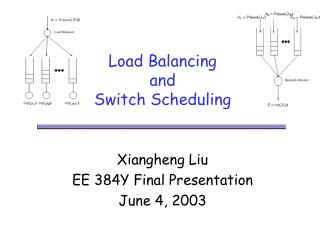Download PresentationLoad Balancing and Switch SchedulingLoad Balancing and Switch Scheduling - PowerPoint PPT Presentation

Download PresentationLoad Balancing and Switch Scheduling
An Image/Link below is provided (as is) to download presentation

Download Policy: Content on the Website is provided to you AS IS for your information and personal use and may not be sold / licensed / shared on other websites without getting consent from its author. While downloading, if for some reason you are not able to download a presentation, the publisher may have deleted the file from their server.

- - - - - - - - - - - - - - - - - - - - - - - - - - - E N D - - - - - - - - - - - - - - - - - - - - - - - - - - -
Presentation Transcript

1. Load Balancing and Switch Scheduling Xiangheng Liu EE 384Y Final Presentation June 4, 2003

2. Motivation – Duality? • Load Balancing Algorithms • Join the Shortest Queue (SQ) • Join a random queue (RAND) • Join the Shortest Queue of Randomly Chosen d queues (SQ(d)) • Randomization with Memory (SQ(d,m)) • Bandwidth Allocation Algorithms • Longest Queue First (LQF) • Serve a random queue (RAND) • Serve the Longest Queue of Randomly chosen d queues (LQF(d)) • Randomization with Memory (LQF(d,m))

3. One Dimensional Case • Queue Dynamics • Linear Approximation • Taking the negative • Let we have

4. Intuition for the Resemblance • Bandwidth allocation  Load balancing • Trick: Negative dual system • Cast bandwidth allocation as load balancing • Reverse the directions of all arrows • Arrivals: arrival of tokens = departure of packets • System state = Number of tokens (Note: the state is always less or equal to 0) • Service: departure of tokens = arrival of packet • SQ in load balancing will translate to LQF in bandwidth allocation based on this duality. • Duality only true for linear approximation.

5. 2-D: N x N crossbar switch • One arrival at a given input per time slot. • No buffering at the input. • Each block can only read from one input per time slot.

6. Dual of MWM in Load Balancing • Load balancing in crossbar switches is essentially a matching problem. • Switch scheduling is better studied in 2-D. • Cast load balancing as switch scheduling. • Design load balancing algorithm based on MWM scheduling. • At time n, if there is no packet arriving at input i, we let qij =  for all j = 1, …, N. • Find a matching that minimizes the weighted sum of queue lengths.

7. Poisson(n) Load Balancer Bandwidth Allocator exp(n) Joint Load Balancing and Switch Scheduling: 1-D • Dual algorithms usually give optimal solutions for linear systems. • Kalman filter and State feedback controller • Linear Modulation and Matched filter.

8. Mean Field Analysis • Assume the load balancer uses SQ(d) and the bandwidth allocation adopts LQF(d). • si(t) – fraction of queues with load at least i. • Mean Field Analysis – differential equation • In Equilibrium, • Backward Recursion:

9. Performance Comparison

10. Entropy Rate of Load Balancing System • Entropy rate of one dimensional load balancing system with a class of randomized algorithm. • Theorem 1 [Nair, et. Al. 2001] Under mild conditions, the entropy rate of queue size process of the load balancing system with any algorithm describable by the coin toss model is equal to (HER(A) + H(S) + H(C)). • Same class of randomized algorithm exists for bandwidth allocation. • If the queue dynamics were linear, the dual bandwidth allocation system should have the same entropy rate.

11. Dual Analysis – Entropy rate of randomized bandwidth allocation • Failed Attempt – try to prove the two systems have equal entropy rates. • Conjecture 1 – The dual bandwidth allocation system has an entropy rate that is upper bounded by (HER(A) + H(S) + H(I) + H(C)) and lower bounded by (HER(A) + H(S+I)). • The bijection that proves Theorem 1 for load balancing is not true for switch scheduling. • Reason – the server may choose an empty queue with the result of coin tosses and it will be tossed again in the next time slot.

12. Conclusions • The Load Balancing and Switch Scheduling are duals of each other if we assume the linear queue dynamics approximation. • We can design new algorithms in one area by taking advantage of the research in the other. • Joint load balancing algorithms lead to great performance gains over the system where only load balancing is used. • Failed attempt – relate the entropy rates of the dual systems.